Next: MINIMUM COLOR SUM Up: Covering and Partitioning Previous: MINIMUM INDEPENDENT DOMINATING SET   Index

### MINIMUM GRAPH COLORING

• INSTANCE: Graph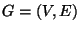.
• SOLUTION: A coloring of G, i.e., a partition of V into disjoint sets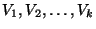such that eachis an independent set for G.
• MEASURE: Cardinality of the coloring, i.e., the number of disjoint independent sets.

• Good News: Approximable within.
• Bad News: Not approximable within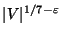for any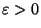.
• Comment: Also called Minimum Chromatic Number. Not approximable withinfor any, unless ZPP=NP . If the graph is 3-colorable, the problem is approximable within, but not approximable within. If the graph is k-colorable the problem is approximable within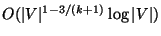. Approximable with an absolute error guarantee of 1 on planar graphs by the Four Color Theorem. Approximable within 3 but not within 1.33 for unit disk graphs . Approximable within 1+1/e + o(1) on circular arc graphs . Approximable within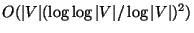in hypergraphs , and is hard to approximate withinunless NP=ZPP [257,340]. MINIMUM COLORING WITH DEFECT D, the variation where the color classesmay induce graphs of degree at most d, is not approximable withinfor some. MINIMUM FRACTIONAL CHROMATIC NUMBER, the linear programming relaxation in which the independent setsdo not need to be disjoint, and in the solution every independent setis assigned a nonnegative value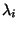such that for each vertexthe sum of the values assigned to the independent sets containing v is at most 1, and the measure is the sum, is always withinfactor from the chromatic number thus the same bad news apply . The complementary maximization problem, where the number of not needed colors'', i.e.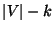, is to be maximized, is approximable within 360/289 .
• Garey and Johnson: GT4Next: MINIMUM COLOR SUM Up: Covering and Partitioning Previous: MINIMUM INDEPENDENT DOMINATING SET   Index
Viggo Kann
2000-03-20